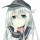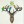# 『数据结构』RMQ 问题

RMQ (Range Minimum/Maximum Query)，即区间最值问题。

## 相关算法

1. 朴素（搜索），时间复杂度： $O(n)-O(q×n)$ ，online
2. 线段树，时间复杂度： $O(n)-O(q×\log n)$ ，online
3. ST（动态规划），时间复杂度： $O(n×\log n)-O(q)$ ，online
4. RMQ标准算法，先规约为 LCA ，再规约成约束 RMQ ，时间复杂度： $O(n)-O(q)$ ，online

## ST 算法

dp[*] dp[*] dp[*] dp[*] dp[*] dp[*] dp[*] dp[*]
dp[*] 1
dp[*] 1 1 1 5 2
dp[*] 3 1 1 5 7 6 2
dp[*] 4 3 1 5 7 8 6 2

### 预处理

void ST_Init(const vector<int> &A)
{
int n=A.size();
for(int i=0; i<n; i++)
dp[i]=A[i];
for(int j=1; (1<<j)<=n; j++)
for(int i=0; i+(1<<j)<=n; i++)
dp[i][j]=min(dp[i][j-1],dp[i+(1<<(j-1))][j-1]);
}


### 查询

int RMQ(int L,int R)
{
int k=0;
while((1<<(k+1))<=R-L+1)k++;
return min(dp[L][k],dp[R-(1<<k)+1][k]);
}


## 线段树

[2,6] 这一个大区间分解为不相交的三个小区间 [2,3]、[4,5]、 ，而最终的结果便由这三个节点中所维护的信息决定咯！## RMQ 标准算法

### 7 只已被捕捉

•cc1Wall Apple Safari | 605.1.15 Mac OS X

棒棒！网站优秀

•banxia Chrome | 59.0.3071.92 Linux

你依然可以在我这里寻找图片的说。

•千千 Chrome | 60.0.3107.4 Windows 10

被发现了，怎么办，好紧张o(￣ヘ￣o＃) 会不会怪罪千千，呀！（逃

•banxia Chrome | 59.0.3071.92 Linux

依然可以的说，不介意的。我在Pixiv找图片也找了蛮久的。•千千 Chrome | 60.0.3107.4 Windows 10

以后我也在 Pixiv 上找找，谢谢啦~•晴和君 Apple Safari | 603.2.4 Mac OS X

虽然学过资料结构，但是都忘了•千千 Chrome | 60.0.3107.4 Windows 10

既然学过，再看的话就很轻松啦~